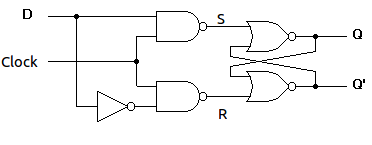# Q.no 11

## D-Type Flip-Flop

A D flip-flop is widely used as the basic building block of random access memory (RAM) and registers.

A D flip flop has a single data input. This type of flip flop is obtained from the SR flip flop by connecting the R input through an inverter, and the S input is connected directly to data input. The modified clocked SR flip-flop is known as D-flip-flop and is shown below. From the truth table of SR flip-flop we see that the output of the SR flip-flop is in unpredictable state when the inputs are same and high. In many practical applications, these input conditions are not required. These input conditions can be avoided by making them complement of each other.Figure-3:Circuit diagram of D flip flop

Characteristic table :Characteristic Equation:

Qn+1    =DQn +DQ=D(Qn + Qn)

Qn+1    =D

Exicitation Table:# Q.No 12## Q.no 13